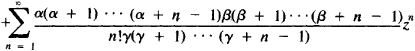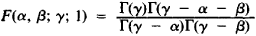# Hypergeometric Series

Also found in: Wikipedia.

## hypergeometric series

[‚hī·pər‚jē·ə′me·trik ′sir·ēz]
(mathematics)
A particular infinite series which in certain cases is a solution to the hypergeometric equation, and having the form:
McGraw-Hill Dictionary of Scientific & Technical Terms, 6E, Copyright © 2003 by The McGraw-Hill Companies, Inc.
The following article is from The Great Soviet Encyclopedia (1979). It might be outdated or ideologically biased.

## Hypergeometric Series

a series of the form

F(α, β; γ; z) = 1The hypergeometric series was studied for the first time by L. Euler in 1778. Expansions of many functions into infinite series are special cases of hypergeometric series. For example,The hypergeometric series has meaning if γ is not equal to zero or a negative integer. It converges at |z| < 1. If, in addition, γ - α - β > 0, then the hypergeometric series also converges for z = 1. In this case, Gauss’ formula is valid:where Γ(z) is the gamma function. An analytic function, defined for |z| < 1 by a hypergeometric series is called a hypergeometric function and plays an important role in the theory of differential equations.

References in periodicals archive ?
Gauss hypergeometric function and confluent hypergeometric function are special cases of the generalized hypergeometric series [sub.p][F.sub.q](p, q [member of] N) defined as (see [8, p.73]) and [9, pp.
Karlsson, Multiple Gaussian hypergeometric series, Ellis Horwood Series: Mathematics and its Applications, Ellis Horwood Ltd., Chichester; Halsted Press [John Wiley & Sons, Inc.], New York, NY, USA, 1985.
The quantum operators are widely used in mathematic fields such as hypergeometric series, complex analysis, orthogonal polynomials, combinatorics, hypergeometric functions, and the calculus of variations.
of the generalized hypergeometric series (the both sides of this equality are rational functions of [a.sub.1], [a.sub.2], [b.sub.1], [b.sub.2]), and the expression
Other examples achieved by termination of the hypergeometric series expansions of the functions of the Heun class include the recently reported inverse square root , Lambert-W step , and Lambert-W singular  potentials.
Wenchang, "Inversion Techniques and Combinatorial Identities: Balanced Hypergeometric Series," Rocky Mountain J.
This paper presents a new method for finding identities for hypergeometric series, covering the Appell-Lauricella hypergeometric series, the (Gauss) hypergeometric series, and the generalized hypergeometric series.
Rahman, Basic Hypergeometric Series (with a Foreword by Richard Askey), Encyclopedia of Mathematics and Its Applications, 35, Cambridge University Press, Cambridge, New York, Port Chester, Melbourne and Sydney, 1990; Second edition, Encyclopedia of Mathematics and Its Applications, 96, Cambridge University Press, Cambridge, London and New York, 2004.
We now give the definition of the hypergeometric series which will be used in obtaining some integrals.
This led to remarkable discovery of Heines formula for a q-hypergeometric function as a generalization of the hypergeometric series and its connection to the Ramanujan product formula, relation between Eulers identities and the Jacobi Triple product identity in the 19th century.
Since the hypergeometric series in (83) converges absolutely in D, it follows that F([beta], [gamma], [delta]; z) defines an analytic function in D and plays an important role in the theory of univalent functions.

Site: Follow: Share:
Open / Close